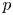Home

### Diophantine equations (11Dxx)

• Article
• ##### Fermat’s Last Theorem over$\mathbb {Q}(\sqrt {\text{5}})$ and$\mathbb {Q}(\sqrt {\text{17}})$
• Canadian Journal of Mathematics, First View

### Forms and linear algebraic groups (11Exx)

• Article
• ##### COHOMOLOGY OF THE BRUHAT–TITS STRATA IN THE UNRAMIFIED UNITARY RAPOPORT–ZINK SPACE OF SIGNATURE$(1,n-1)$
• Nagoya Mathematical Journal, First View

### Discontinuous groups and automorphic forms (11Fxx)

• Article
• ##### A$p$-adic monodromy theorem for de Rham local systems
• Compositio Mathematica, Volume 158, Issue 12

• Article
• ##### Fermat’s Last Theorem over$\mathbb {Q}(\sqrt {\text{5}})$ and$\mathbb {Q}(\sqrt {\text{17}})$
• Canadian Journal of Mathematics, First View

### Diophantine approximation, transcendental number theory (11Jxx)

• Article
• ##### Rational points on nonlinear horocycles and pigeonhole statistics for the fractional parts of$\sqrt {n}$
• Ergodic Theory and Dynamical Systems, First View

### Multiplicative number theory (11Nxx)

• Article
• ##### A dynamical approach to the asymptotic behavior of the sequence$\Omega (n)$
• Ergodic Theory and Dynamical Systems, First View

### Additive number theory; partitions (11Pxx)

• Article
• ##### Rational points on nonlinear horocycles and pigeonhole statistics for the fractional parts of$\sqrt {n}$
• Ergodic Theory and Dynamical Systems, First View

### Algebraic number theory: local and $p$-adic fields (11Sxx)

• Article
• ##### Elements of prime order in Tate–Shafarevich groups of abelian varieties over${\mathbb Q}$
• Forum of Mathematics, Sigma, Volume 10

• Article
• ##### Sum expressions for Kubota–Leopoldt $p$-adic $L$-functions
• Proceedings of the Edinburgh Mathematical Society, Volume 65, Issue 2

### Connections with logic (11Uxx)

• Article
• ##### WAVE FRONT HOLONOMICITY OF$\text{C}^{\text{exp}}$-CLASS DISTRIBUTIONS ON NON-ARCHIMEDEAN LOCAL FIELDS
• Forum of Mathematics, Sigma, Volume 8

### Computational number theory (11Yxx)

• Article
• ##### Sum expressions for Kubota–Leopoldt $p$-adic $L$-functions
• Proceedings of the Edinburgh Mathematical Society, Volume 65, Issue 2